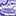Announcements
The way to achieve your own success is the willingness to help somebody else. Go for it!
cancel
Showing results for
Did you mean:Contributor III

## Format pattern with conditional decimalThe image above shows my current display in a chart, currently setup as Expression Default in the Chart Properties (Number tab).  I would like to only show up to two decimal places when the decimal is not 0.  Ideally I would like to see:

3123.89

31911.27

450

72.42

1149.38

19468.14

471.25

14882.25

10428.75

14006.5

If I try to force the format pattern "Fixed to 2 decimals", it always shows .00 on the whole numbers and .#0 on the values that have only one decimal place.  Some of the decimals are very large, which is why I want to only show 2 decimal places.  If I use the round() function, it automatically tacks on two decimal places, even on whole numbers, so I'm still seeing .00 on values like 450 above.

Is it possible in Qlik to only show a certain number of decimal places, but only when they are non-zero?

1 Solution

Accepted SolutionsPartner - Champion III

Sunny, I think you can make it even simpler. The idea is to set the Number format to Expression Default, and use that one whenever the fraction has 2 digits or less. More digits will use a num() with a specific number format string. This translates into something like:

=IF(Len(Trim(SubField(NumberField,'.',2))) > 2,Num(NumberField,'#0.00'),NumberField)

It even works in a text box that has no selection for expression default. Try it with a variable instead of NumberField and set the variable to different numbers.

3 RepliesMVP

Try this may be:

=If(Len(Trim(SubField(NumberField, '.', 2))) > 2, Num(NumberField, '#,##0.00'),

If(Len(Trim(SubField(NumberField, '.', 2))) = 1, Num(NumberField, '#,##0.0'), Num(NumberField, '#,##0')))Partner - Champion III

Sunny, I think you can make it even simpler. The idea is to set the Number format to Expression Default, and use that one whenever the fraction has 2 digits or less. More digits will use a num() with a specific number format string. This translates into something like:

=IF(Len(Trim(SubField(NumberField,'.',2))) > 2,Num(NumberField,'#0.00'),NumberField)

It even works in a text box that has no selection for expression default. Try it with a variable instead of NumberField and set the variable to different numbers.MVP

Make senseCommunity Browser